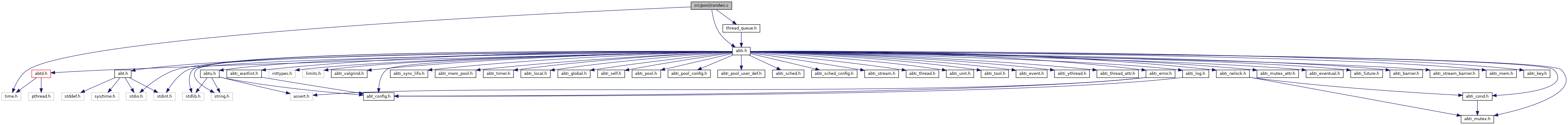ARGOBOTS  dce6e727ffc4ca5b3ffc04cb9517c6689be51ec5
randws.c File Reference
`#include "abti.h"`
`#include "thread_queue.h"`
`#include <time.h>`
Include dependency graph for randws.c:Go to the source code of this file.

struct  data

## Macros

#define POOL_CONTEXT_POP_TAIL   (ABT_POOL_CONTEXT_OWNER_SECONDARY)

## Typedefs

typedef struct data data_t

## Functions

static int pool_init (ABT_pool pool, ABT_pool_config config)

static void pool_free (ABT_pool pool)

static ABT_bool pool_is_empty (ABT_pool pool)

static size_t pool_get_size (ABT_pool pool)

static void pool_push_shared (ABT_pool pool, ABT_unit unit, ABT_pool_context context)

static void pool_push_private (ABT_pool pool, ABT_unit unit, ABT_pool_context context)

static ABT_thread pool_pop_shared (ABT_pool pool, ABT_pool_context context)

static ABT_thread pool_pop_private (ABT_pool pool, ABT_pool_context context)

static ABT_thread pool_pop_wait (ABT_pool pool, double time_secs, ABT_pool_context context)

static void pool_push_many_shared (ABT_pool pool, const ABT_unit *units, size_t num_units, ABT_pool_context context)

static void pool_push_many_private (ABT_pool pool, const ABT_unit *units, size_t num_units, ABT_pool_context context)

static void pool_print_all (ABT_pool pool, void *arg, void(*print_fn)(void *, ABT_thread))

static void pool_free_unit (ABT_pool pool, ABT_unit unit)

static int pool_remove_shared (ABT_pool pool, ABT_unit unit)

static int pool_remove_private (ABT_pool pool, ABT_unit unit)

static ABT_unit pool_pop_timedwait (ABT_pool pool, double abstime_secs)

static ABT_bool pool_unit_is_in_pool (ABT_unit unit)

static data_tpool_get_data_ptr (void *p_data)

ABTU_ret_err int ABTI_pool_get_randws_def (ABT_pool_access access, ABTI_pool_required_def *p_required_def, ABTI_pool_optional_def *p_optional_def, ABTI_pool_deprecated_def *p_deprecated_def)

## ◆ POOL_CONTEXT_POP_TAIL

 #define POOL_CONTEXT_POP_TAIL   (ABT_POOL_CONTEXT_OWNER_SECONDARY)

Definition at line 49 of file randws.c.

Value:

Definition at line 45 of file randws.c.

## ◆ data_t

 typedef struct data data_t

Definition at line 55 of file randws.c.

## ◆ ABTI_pool_get_randws_def()

 ABTU_ret_err int ABTI_pool_get_randws_def ( ABT_pool_access access, ABTI_pool_required_def * p_required_def, ABTI_pool_optional_def * p_optional_def, ABTI_pool_deprecated_def * p_deprecated_def )

Definition at line 64 of file randws.c.

Referenced by ABTI_pool_create_basic().

## ◆ pool_create_unit()

static

Definition at line 403 of file randws.c.

Referenced by ABTI_pool_get_randws_def().

## ◆ pool_free()

 static void pool_free ( ABT_pool pool )
static

Definition at line 134 of file randws.c.

Referenced by ABTI_pool_get_randws_def().

## ◆ pool_free_unit()

 static void pool_free_unit ( ABT_pool pool, ABT_unit unit )
static

Definition at line 410 of file randws.c.

Referenced by ABTI_pool_get_randws_def().

## ◆ pool_get_data_ptr()

 static data_t* pool_get_data_ptr ( void * p_data )
inlinestatic

Definition at line 57 of file randws.c.

## ◆ pool_get_size()

 static size_t pool_get_size ( ABT_pool pool )
static

Definition at line 149 of file randws.c.

Referenced by ABTI_pool_get_randws_def().

## ◆ pool_init()

 static int pool_init ( ABT_pool pool, ABT_pool_config config )
static

Definition at line 112 of file randws.c.

Referenced by ABTI_pool_get_randws_def().

## ◆ pool_is_empty()

 static ABT_bool pool_is_empty ( ABT_pool pool )
static

Definition at line 142 of file randws.c.

Referenced by ABTI_pool_get_randws_def().

## ◆ pool_pop_many_private()

 static void pool_pop_many_private ( ABT_pool pool, ABT_thread * threads, size_t max_threads, size_t * num_popped, ABT_pool_context context )
static

Definition at line 337 of file randws.c.

Referenced by ABTI_pool_get_randws_def().

## ◆ pool_pop_many_shared()

 static void pool_pop_many_shared ( ABT_pool pool, ABT_thread * threads, size_t max_threads, size_t * num_popped, ABT_pool_context context )
static

Definition at line 309 of file randws.c.

Referenced by ABTI_pool_get_randws_def().

## ◆ pool_pop_private()

 static ABT_thread pool_pop_private ( ABT_pool pool, ABT_pool_context context )
static

Definition at line 296 of file randws.c.

Referenced by ABTI_pool_get_randws_def().

## ◆ pool_pop_shared()

 static ABT_thread pool_pop_shared ( ABT_pool pool, ABT_pool_context context )
static

Definition at line 277 of file randws.c.

Referenced by ABTI_pool_get_randws_def().

## ◆ pool_pop_timedwait()

 static ABT_unit pool_pop_timedwait ( ABT_pool pool, double abstime_secs )
static

Definition at line 255 of file randws.c.

Referenced by ABTI_pool_get_randws_def().

## ◆ pool_pop_wait()

 static ABT_thread pool_pop_wait ( ABT_pool pool, double time_secs, ABT_pool_context context )
static

Definition at line 222 of file randws.c.

Referenced by ABTI_pool_get_randws_def().

## ◆ pool_print_all()

 static void pool_print_all ( ABT_pool pool, void * arg, void(*)(void *, ABT_thread) print_fn )
static

Definition at line 377 of file randws.c.

Referenced by ABTI_pool_get_randws_def().

## ◆ pool_push_many_private()

 static void pool_push_many_private ( ABT_pool pool, const ABT_unit * units, size_t num_units, ABT_pool_context context )
static

Definition at line 205 of file randws.c.

Referenced by ABTI_pool_get_randws_def().

## ◆ pool_push_many_shared()

 static void pool_push_many_shared ( ABT_pool pool, const ABT_unit * units, size_t num_units, ABT_pool_context context )
static

Definition at line 184 of file randws.c.

Referenced by ABTI_pool_get_randws_def().

## ◆ pool_push_private()

 static void pool_push_private ( ABT_pool pool, ABT_unit unit, ABT_pool_context context )
static

Definition at line 171 of file randws.c.

Referenced by ABTI_pool_get_randws_def().

## ◆ pool_push_shared()

 static void pool_push_shared ( ABT_pool pool, ABT_unit unit, ABT_pool_context context )
static

Definition at line 156 of file randws.c.

Referenced by ABTI_pool_get_randws_def().

## ◆ pool_remove_private()

 static int pool_remove_private ( ABT_pool pool, ABT_unit unit )
static

Definition at line 369 of file randws.c.

Referenced by ABTI_pool_get_randws_def().

## ◆ pool_remove_shared()

 static int pool_remove_shared ( ABT_pool pool, ABT_unit unit )
static

Definition at line 358 of file randws.c.

Referenced by ABTI_pool_get_randws_def().

## ◆ pool_unit_is_in_pool()

 static ABT_bool pool_unit_is_in_pool ( ABT_unit unit )
static

Definition at line 396 of file randws.c.

Referenced by ABTI_pool_get_randws_def().